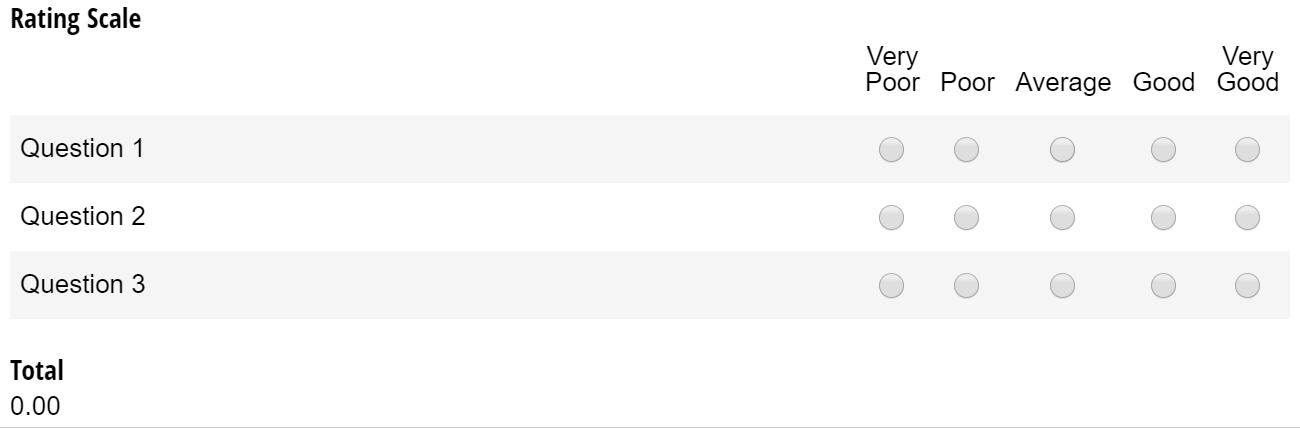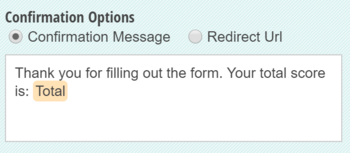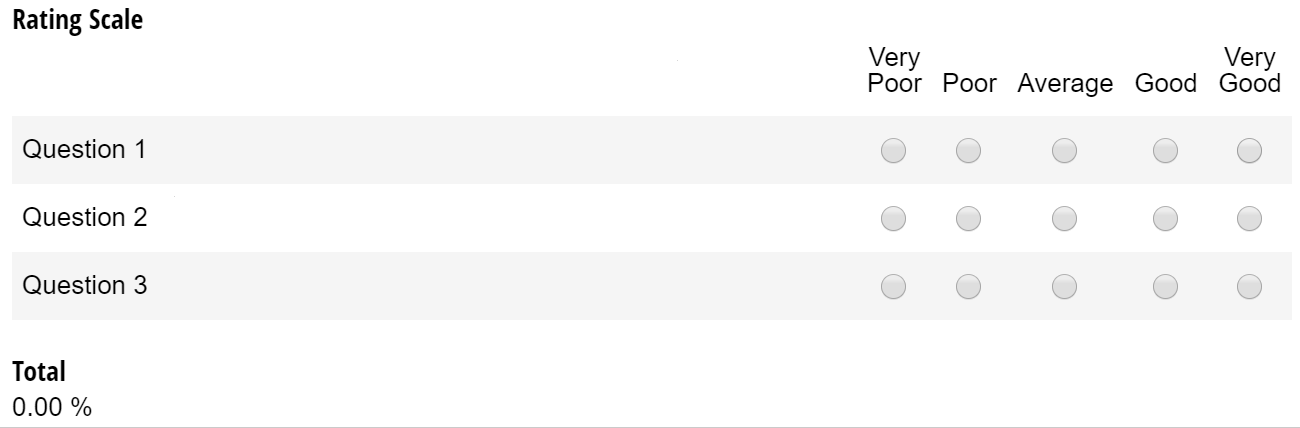# 3 Easy Ways to Calculate Rating Scales!By Nadia A. | July 14, 2017

Whether you’re soliciting customer feedback or creating a personality quiz, rating scales (aka Likert sales) are one of the best methods for collecting a broad range of opinions and behaviors.

In Cognito Forms, you can easily add either a predefined (Satisfied/Unsatisfied, Agree/Disagree, etc.) or completely custom rating scale to your form. Every question in a rating scale has an internal numerical value based on the number of rating options; for example, on a Good/Poor rating scale, Very Poor has a value of 1, while Very Good has a value of 5. You can reference these values to calculate scores, percentages, and more!

Feel free to test out these calculations above, or save this form into your organization.

## 1. Total score

The easiest way to calculate a rating scale is to simply add up the total score. To do this, start by adding a Calculation field to your form, and make sure that it’s set to internal view only.

Next, target your individual rating scale questions by entering the name of your rating scale, the rating scale question, and “_Rating”:

`=RatingScale.Question1_Rating + RatingScale.Question2_Rating + RatingScale.Question3_Rating`

And that’s it! Now, the value of each question will be summed up:If you want to display the total to your users, just insert the Calculation field into your form’s confirmation message or confirmation email using the Insert Field option:## 2. Weighted score

Calculating a total score is simple enough, but there are a ton of other functions you can perform using rating scale values. For example, divide the rating scale total by the number of questions to calculate the average:

`=(RatingScale.Question1_Rating + RatingScale.Question2_Rating + RatingScale.Question3_Rating) / 3`

Or, if you have multiple rating scales, you can average each one and add them together:

`=((RatingScale.Question1_Rating + RatingScale.Question2_Rating + RatingScale.Question3_Rating) / 3) + ((RatingScale2.Question1_Rating + RatingScale2.Question2_Rating + RatingScale2.Question3_Rating) / 3)`

If you want the average of one rating scale to weigh more than another, just multiply each rating scale average by the percentage that it’s worth (in this case, 40%):

`=(((RatingScale.Question1_Rating + RatingScale.Question2_Rating + RatingScale.Question3_Rating) / 3) *.4)`

## 3. Percentages

Rather than displaying a total in points, you could also calculate a total percentage. To do this, add a Calculation field to your form, and set it to the Percent type. Next, write an expression that calculates the average of the rating scale divided by the number of possible options. For example, if your rating scale has three questions, and five options to choose from:

`=((RatingScale.Question1_Rating + RatingScale.Question2_Rating + RatingScale.Question3_Rating) / 3) /5`

Now, the total percentage will be calculated based on a 100 point scale: